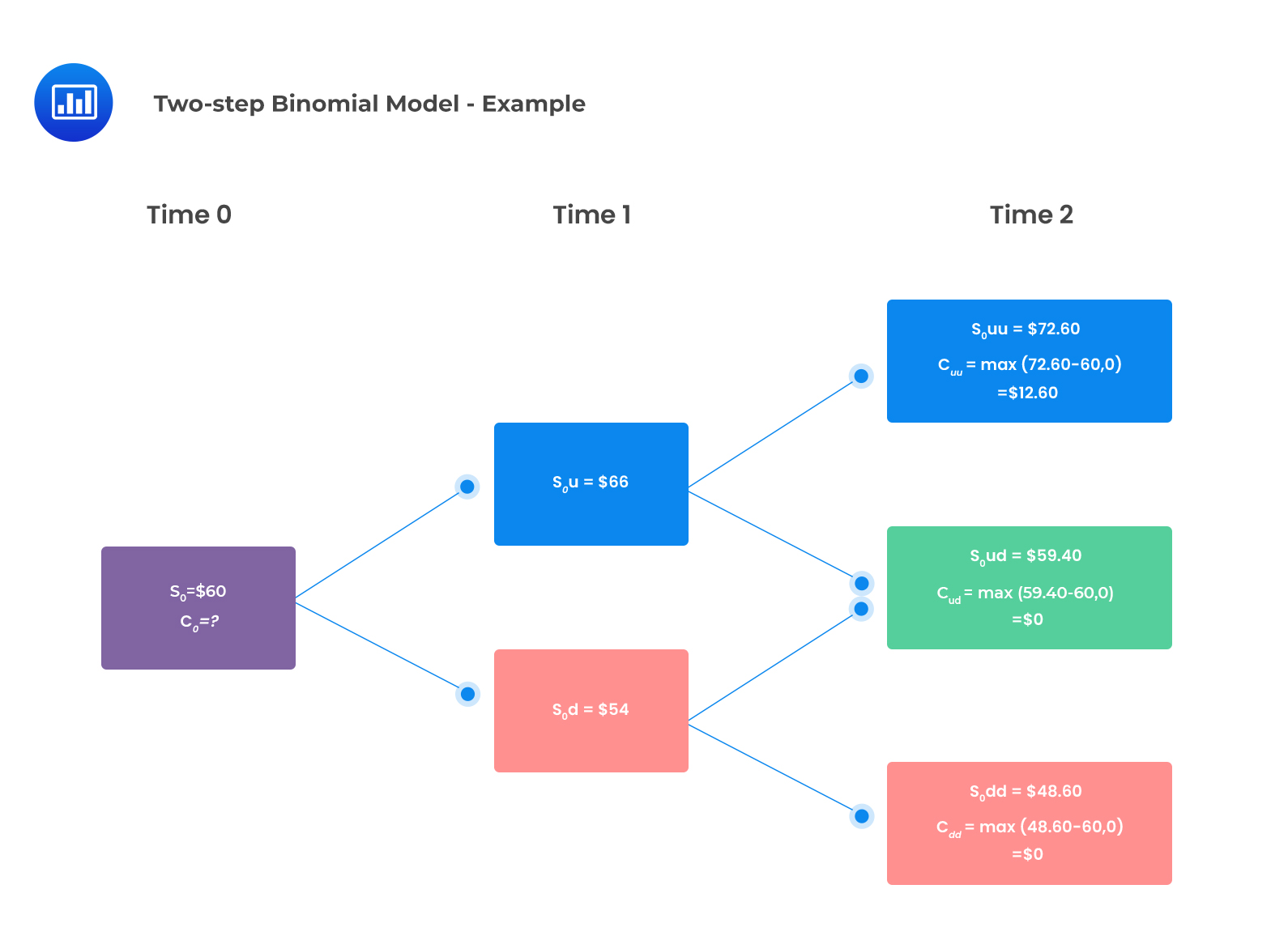# Expectations Valuation Approach

## One-Step Binomial Tree

The idea that a hedged portfolio returns the risk-free rate can determine the initial value of a call or put. The expectations approach calculates the values of the option by taking the present value of the expected terminal option payoffs. This approach utilizes risk-neutral probabilities instead of true probabilities.

Thus, the initial value of a call and put respectively are determined using the following formulas:

$$c_{0}=\frac{qc_{u}+(1-q)c_{d}}{1+r}$$

And

$$p_{0}=\frac{qp_{u}+(1-q)p_{d}}{1+r}$$

Where:

$$q=\frac{(1+r)-d}{u-d}$$

$$r$$ is the risk-free rate for a single period.

$$q$$ gives the risk-neutral probability of an upward move in price, and $$(1-q)$$ gives the probability of a downward move.

### Example: Expectations Approach for One-Step Binomial Tree

Consider a stock that is currently trading at $50. Assume that the up jump and down jump factors for the stock price are u = 1.20 and d = 0.80. The risk-free rate compounded periodically is 4%. Given a strike price of$50, we can use a single period binomial model to price European call and put options.

#### Price of a European Call Option

Recall:

$$c_{T}=max(S_{T}-K,0)$$

$$S_{0}u=50\times1.20=60$$

$$S_{0}d=50\times0.80=40$$

$$c_{u}=max(60-50,0)=10$$

$$c_{d}=max(40-50,0)=0$$

The value of the call option can then be determined using the formula:

$$c_{0}=\frac{qc_{u}+(1-q)c_{d}}{1+r}$$

Where:

\begin{align*}q&=\frac{(1+r)-d}{u-d}\\&=\frac{(1.04)-0.8}{1.20-0.80}\\&=0.6\end{align*}

$$c_{0}=\frac{0.6\times10+(1-0.6)\times0}{1.04}=5.77$$

#### Price of a European Put Option

$$p_{T}=max(K-S_{T},0)$$

$$p_{u}=max(50-60,0)=0$$

$$p_{d}=max(50-40,0)=10$$

\begin{align*}p_{0}&=\frac{qp_{u}+(1-q)p_{d}}{1+r}\\&=\frac{0.60\times0+(1-0.60)\times10}{1.04}\\&=3.85\end{align*}

## Two-step binomial tree

The expectations approach can also be applied to the two-step binomial model to determine the value of options.

Let q to be the risk-neutral probability of an up move, the price of a European call option can be determined using the two-step binomial model:

$$c_{o}=\frac{q^{2}c_{uu}+2q(1-q)c_{ud}+(1-q)^{2}c_{dd}}{(1+r)^2}$$

The two-period European put value is given as:

$$p_{0}=\frac{q^{2}p_{uu}+2q(1-q)p_{ud}+(1-q)^{2}p_{dd}}{(1+r)^{2}}$$

### Example: Expectations Approach for two-Step Binomial Model

Suppose you have a stock that is currently trading at $60. A two-year European call option on the stock is available with a strike price of$60. The risk-free rate of 2% per annum. Given that the up-move factor is 1.10 and the down-move factor is 0.90, the value of the call option using a two-period binomial model is closest to:

### Solution

The risk-neutral probability of an up-move is given by:

\begin{align*}q&=\frac{(1+r)-d}{u-d}\\&=\frac{1.02-0.90}{1.1-0.9}\\&=0.6\end{align*}

The probability of down move $$(1-q) = 1-0.6 = 0.4$$

The two-period binomial tree is shown below:The two-period binomial value of the call option:

\begin{align*}c_{o}&=\frac{q^{2}c_{uu}+2q(1-q)c_{ud}+(1-q)^{2}c_{dd}}{(1+r)^{2}}\\&=\frac{0.6^{2}\times12.60+2\times0.6\times0.4\times0\times0.4^{2}\times0}{(1.02)^{2}}\\&=4.36\end{align*}

## Question

Nabi Gudka, CFA, applies the expectations approach to value a European call option on the common shares of Wipro Inc.

The expectation approach most likely utilizes:

1. A risk premium for discounting
2. Risk-neutral probabilities
3. Actual probabilities

### Solution

Under the expectations approach, the expected future payoff is calculated using risk-neutral probabilities, and the expected payoff is discounted at the risk-free rate.

Reading 38: Valuation of Contingent Claims

LOS 38 (e): Describe how the value of a European option can be analyzed as the present value of the option’s expected payoff at expiration;

Shop CFA® Exam Prep

Offered by AnalystPrepLevel I
Level II
Level III
All Three Levels
Featured Shop FRM® Exam PrepFRM Part I
FRM Part II
FRM Part I & Part II
Learn with Us

Subscribe to our newsletter and keep up with the latest and greatest tips for success
Shop Actuarial Exams PrepExam P (Probability)
Exam FM (Financial Mathematics)
Exams P & FMGMAT® Complete CourseDaniel Glyn
2021-03-24
I have finished my FRM1 thanks to AnalystPrep. And now using AnalystPrep for my FRM2 preparation. Professor Forjan is brilliant. He gives such good explanations and analogies. And more than anything makes learning fun. A big thank you to Analystprep and Professor Forjan. 5 stars all the way!michael walshe
2021-03-18
Professor James' videos are excellent for understanding the underlying theories behind financial engineering / financial analysis. The AnalystPrep videos were better than any of the others that I searched through on YouTube for providing a clear explanation of some concepts, such as Portfolio theory, CAPM, and Arbitrage Pricing theory. Watching these cleared up many of the unclarities I had in my head. Highly recommended.Nyka Smith
2021-02-18
Every concept is very well explained by Nilay Arun. kudos to you man!2021-02-13